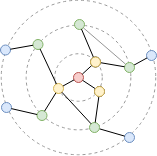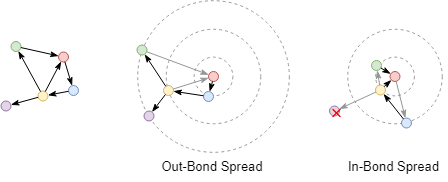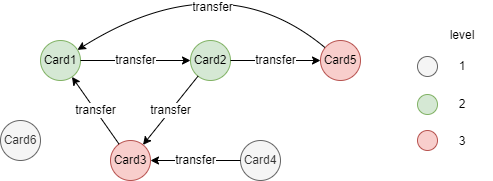# Change Nickname

Current Nickname:

Certifications

Certificate Issued at Valid until Serial No. File
Serial No. Valid until

Not having one? Apply now! >>>

Invoice

ProductName CreateTime ID Price File
ProductName CreateTime ID Price File

# K-Hop All

## Overview

K-Hop Whole Graph algorithm calculates the number of K-hop neighbors for each node and returns those neighbors. The algorithm is widely used in scenarios such as relationship discovery, impact prediction and friend suggestion and so on.

K-Hop Whole Graph can be viewed as the batch execution of UQL command `khop()`. Although Ultipa has optimized the K-Hop Whole Graph algorithm for high concurrency performance, massive system resources can still be consumed by the algorithm in large graphs (over tens of millions nodes and edges) or graphs with many super nodes. Therefore, it is recommended to avoid performing K-Hop Whole Graph calculation that is too deep.

In a graph which `nodes/edges = 100`, the theoretical computation amount required to query 5-hop neighbors of a node is 105 (10 billion computational complexity) and it would take 100ms; then 1 million seconds (12 days) are needed to complete such query in a graph of 10 million nodes.

## Basic Concept

### BFS

BFS is short for Breadth First Search, together with DFS (Depth First Search), are two the most basic traversal principles. K-Hop query follows BFS principle, which means that when traversing the whole graph from one node, it first traverses all the neighbor nodes of the current node that have not arrived yet, and then traverses the neighbor nodes of these neighbor nodes in turns that have not arrived yet.Starting from the red node and traversing by the BFS principle, the yellow, green, blue nodes are found in succession. Color of these nodes is classified by the distance to the start node, that is, the number of edges of the shortest path(s) from the start node to them. This distance is what the `K` means in K-Hop query, which is the `K`-hop neighbors of the start node.

Once the start node is given, K values of the other nodes in the graph are also determined. In other words, if a node is the 5th level neighbor of the start node, then it is impossible to appear in the 4th, 6th or other levels.

### Direction of K-Hop

K-Hop query in directed graph can be restrained by the direction of the edge where the neighbor is located, this is when some nodes do not have K values and not all nodes are found in the traversal result.Starting from the red node in the directed graph on the left side, and traversing by the outbound edges and inbound edges; two distinct traversal results are obtained, the purple node does not belong to any level when traversing in the inbound direction and its K value does not exist.

## Special Case

### Lonely Node, Disconnected Graph

Lonely node will not appear in any K-Hop query result.

For disconnected graph, only nodes belong to the same connected component with the start node are involved in the K-Hop query result, while other nodes cannot be traversed.

### Self-loop Edge

Each node is traversed only once in K-Hop query, so the self-loop edge of node can be considered invalid.

### Directed Edge

When the K-Hop query is performed without specifying the direction of edges, the direction of edges is ignored, and certain K values are calculated for all nodes in the connected component where that start node is located.

## Results and Statistics

Take the transaction graph below as an example, the color of card represent its level, run the K-Hop Whole Graph algorithm against all nodes:Algorithm results: Calculate 1-hop neighbors for each node and count the maximum level of the results, return `_id`, `value` or `_uuid`, `value` according to the execution method, column `value` includes the aggregative counting result(s) and the number of neighbors

_uuid _id value
1 Card1 3, 3
2 Card2 3, 3
3 Card3 2, 3
4 Card4 3, 1
5 Card5 2, 2
6 Card6 , 0

Algorithm statistics: N/A

## Command and Configuration

• Command: `algo(khop_all)`
• Configurations for the parameter `params()`:
Name Type
Default
Specification
Description
ids / uuids []`_id` / []`_uuid` / / IDs or UUIDs of nodes to be calculated; all nodes to be calculated if not set
k_start int 1 >= 1 The minimum K-Hop query depth, the query depth is [`k_start`, `k_end`], `k_end``k_start`
k_end int 1 >= 1 The maximum K-Hop query depth, the query depth is [`k_start`, `k_end`], `k_end``k_start`
src_include int 0 0 or 1 Include the start node in the result or not, 1 means to include the start node, 0 or not set means to exclude the start node
direction string / in/out, case insensitive Edge direction in the path; direction ignored if not set
node_property []`@<schema>?.<property>` / Numeric node property, LTE needed Node property/properties to be aggregatively counted, schema can be either carried or not; must be used with `aggregate_opt`; results without any specified property will not be aggregatively counted
aggregate_opt []string / max / min / mean / sum / var / dev, case insensitive The method that each specified property of the results to be aggregatively counted; must be used with `node_property` and one property corresponds to one aggregative counting method; max means the maximum, min means the minimum, mean means the average, sum means the sum, var means the variance, and dev means the standard deviation
limit int -1 >=-1 Number of results to return; return all results if sets to -1 or not set

Example: Calculate the number of 2-hop neighbors in the outbound direction of all nodes

``````algo(khop_all).params({
k_start: 2,
k_end: 2,
direction: "out"
}) as k2
return k2
``````

Example: Calculate 2-hop and 3-hop neighbors of nodes UUID = 3,4,5 and aggregatively count the maximum value of property @card.level and sum of property @card.balance of the results, also include the start node (UUID = 3,4,5) in each node's results

``````algo(khop_all).params({
uuids: [3,4,5],
k_start: 2,
k_end: 3,
src_include: 1,
node_property: [@card.level, @card.balance],
aggregate_opt: ["max", "sum"]
}) as k2
return k2
``````

## Algorithm Execution

#### 1. File Writeback

Configuration Data in Each Row
Description
filename_ids `_id`,`_id` Each result takes up one line, the first `_id` in each line represents the node that is calculated, the second `_id` represents the neighbor of the calculated node
filename `_id`,`aggregate_result1`,...,`aggregate_resultN`,`count` `_id` represents the node that is calculated, the next is/are the one or multiple aggregative counting result(s) (`aggregate_result1` ~ `aggregate_resultN`), the last `count` is the number of neighbors

Example: Calculate 2-hop and 3-hop neighbors of all nodes and aggregatively count the maximum value of property @card.level and sum of property @card.balance of the results, write the IDs of neighbors of each node back to file named neighbors, write the aggregative counting results and the number of neighbors of each node back to file named counts

``````algo(khop_all).params({
k_start: 3,
k_end: 3,
node_property: [@card.level, @card.balance],
aggregate_opt: ["max", "sum"]
}).write({
file:{
filename_ids: "neighbors",
filename: "counts"
}
})
``````

#### 2. Property Writeback

Configuration Writeback Content Type Data Type
property Number of neighbors Node property `double`

Example: Calculate 2-hop neighbors of all nodes, write the number of neighbors back to node property named khop2

``````algo(khop_all).params({
k_start: 2,
k_end: 2
}).write({
db:{
property: "khop2"
}
})
``````

#### 3. Statistics Writeback

This algorithm has no statistics.

### Direct Return

Alias Ordinal Type
Description
Column Name
0 []perNode Node and its aggregative counting result(s) and number of neighbors `_uuid`, `value`

Example: Calculate 3-hop neighbors of all nodes and aggregatively count the maximum value of property @card.level and sum of property @card.balance of the results, define algorithm results as alias named nei, and return the results

``````algo(khop_all).params({
k_start: 3,
k_end: 3,
node_property: [@card.level, @card.balance],
aggregate_opt: ["max", "sum"]
}) as nei
return nei
``````

### Streaming Return

Alias Ordinal Type
Description
Column Name
0 []perNode Node and its aggregative counting result(s) and number of neighbors `_uuid`, `value`

Example: Calculate 1-hop to 3-hop neighbors of node UUID = 3 and aggregatively count the maximum value of property @card.level of the results, and return only the aggregative counting result and the number of neighbors

``````algo(khop_all).params({
uuids: ,
k_start: 1,
k_end: 3,
node_property: @card.level,
aggregate_opt: max
}).stream() as results
return results.value
``````

### Real-time Statistics

This algorithm has no statistics.